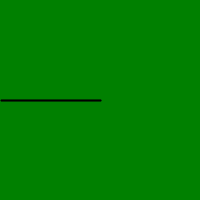Related Articles

# Wand path_start() function in Python

• Last Updated : 11 May, 2020

We can also draw paths in `wand.drawing` module. Each path method expects a destination point, and will draw from the current point to the new point. The destination point will become the new current point for the next applied path method. Paths in wand consist of some other methods to draw different graphics in a path.
In this article we will learn path_start() function. path is initiated using path_start function.

Syntax: wand.drawing.path_start()

Example #1:

 `from` `wand.image ``import` `Image``from` `wand.drawing ``import` `Drawing``from` `wand.color ``import` `Color`` ` `with Drawing() as draw:``    ``draw.stroke_width ``=` `2``    ``draw.stroke_color ``=` `Color(``'black'``)``    ``draw.path_start()``     ` `    ``# Start middle-left``    ``draw.path_move(to ``=``(``100``, ``100``))``    ``draw.path_horizontal_line(``1``)``     ` `    ``# Close first & last points``    ``draw.path_close()``    ``draw.path_finish()``    ``with Image(width ``=` `200``, ``               ``height ``=` `200``,``               ``background ``=` `Color(``'green'``)) as image:``         ` `        ``draw(image)``        ``image.save(filename ``=` `"pathstart.png"``)`

Output:Example #2:

 `from` `wand.image ``import` `Image``from` `wand.drawing ``import` `Drawing``from` `wand.color ``import` `Color``  ` `with Drawing() as draw:``    ``draw.stroke_width ``=` `2``    ``draw.stroke_color ``=` `Color(``'black'``)``    ``draw.path_start()``     ` `    ``# Start middle-left``    ``draw.path_move(to``=``(``100``, ``100``))``     ` `    ``# draw a vertical line from path initial point``    ``draw.path_vertical_line(``1``)``     ` `    ``# Close first & last points``    ``draw.path_close()``    ``draw.path_finish()``    ``with Image(width``=``200``,``               ``height``=``200``,``               ``background``=``Color(``'green'``)) as image:``        ``draw(image)``        ``image.save(filename ``=` `"pathstart.png"``)`

Output:Attention geek! Strengthen your foundations with the Python Programming Foundation Course and learn the basics.

To begin with, your interview preparations Enhance your Data Structures concepts with the Python DS Course. And to begin with your Machine Learning Journey, join the Machine Learning – Basic Level Course

My Personal Notes arrow_drop_up